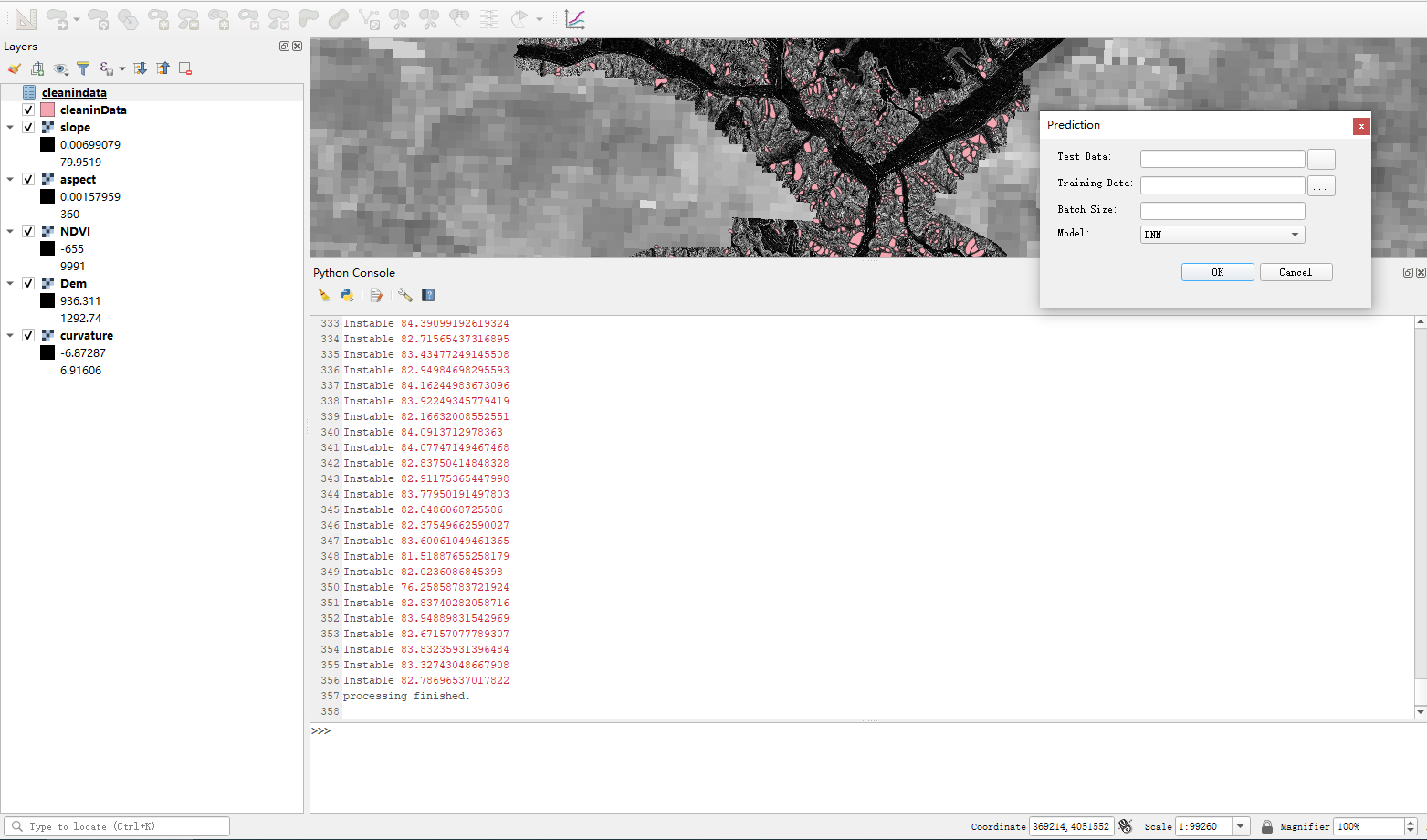# 灾害智能识别-Deep Neural Networks算法

​ 深度神经网络是机器学习(ML, Machine Learning)领域中一种技术，是深度学习的基础。

## 1. 从感知机到神经网络

​ 感知机模型是一个有若干输入和一个输出的模型，如下图: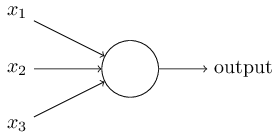​ 输出和输入之间学习到一个线性关系，得到中间输出结果： $z=\sum\limits_{i=1}^mw_ix_i + b$ ​ 然后是激活函数: $sign(z)=\begin{cases}-1 & z<0\\1 & z\geq 0\end{cases}$ ​ 最后得到我们想要的输出结果1或者-1。

​ 感知机模型适用于二元分类，无法学习比较复杂的非线性模型，使用范围有限。神经网络在感知机的模型上进行了扩展：

### 1.1 加入隐藏层

​ 隐藏层可以有多层，增强模型的表达能力。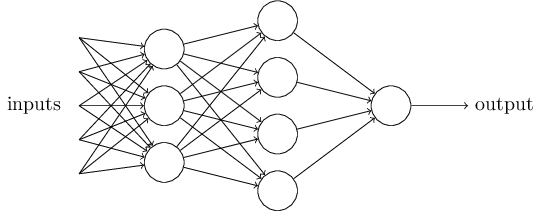### 1.2 输出层的神经元可以有多个输出

​ 模型可以灵活应用于分类回归，以及其他机器学习领域,例如降维和聚类等问题。输出层具有多神经元 ，如下图。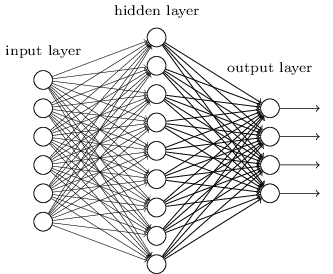### 1.3 扩展激活函数

​ 感知机的激活函数是$sign(z)$,其处理能力有限，神经网络对激活函数进行了扩展，例如逻辑回归使用过的$Sigmoid$函数： $f(z)=\frac{1}{1+e^{-z}}$ ​ 还有后来出现的$tanx$$softmax$,和$ReLU$等。不同的激活函数可以增强神经网络的表达能力。

## 2. DNN的基本结构

​ 神经网络是基于感知机的扩展，而DNN是具有多层隐藏层的神经网络。DNN也叫做多层感知机（Multi-Layer perceptron,MLP）。

​ DNN内部的神经网络层可以分为三类，输入层、隐藏层和输出层，如下图示例，第一层是输入层，最后一层是输出层，中间为隐藏层。​ 层与层之间是全连接的，第$i$层的任意一个神经元一定与第$i+1$层的任意一个神经元相连。其提现了一个基本的线性关系：$z=\sum\limits w_ix_i + b$ + 激活函数$\sigma(z)$。

## 3、灾害智能识别

### 3.1 地质灾害数据清洗与数据抽析。

​ 对不同GIS专题数据进行数据清洗与数据抽析。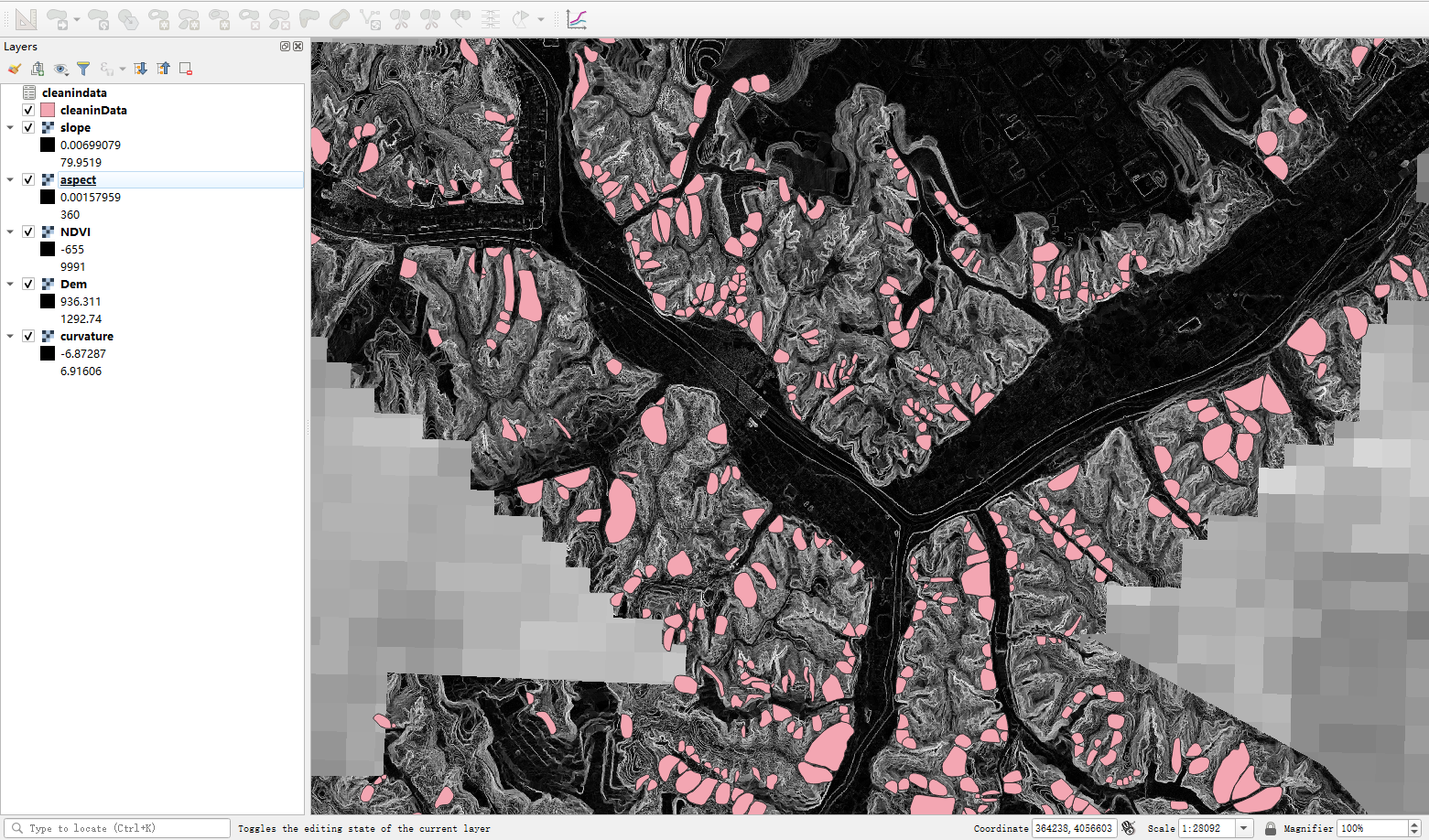### 3.2 地质灾害数据标准化

​ 对清洗后的数据进行数据标准化处理，形成可标准化Input数据。### 3.3 地质灾害数据训练与预测

​ 利用标准化后的Input数据进行训练与预测。